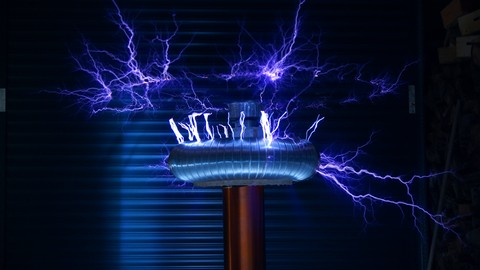# UdemyHigh Voltage and Insulators for Electrical Power EngineeringThis course is the first to introduce and explain High Voltage engineering branch of electrical power engineering

What you'll learn
• Learn the importance and the need for high voltage
• Understand the insulator function for isolation of H.V
• Understand How breakdown occurs in insulator
• Understand the meaning of dielectric strength of insulator
• Realize the different applications of Using high voltage
• Learn different types of testing high voltages in lab
• Understand the concept and operation of HV testing transformer
• Realize the difference between HV testing transformer and Power transformer
• Cascaded HV transformers for higher voltage generation
• Understand the operation of resonant transformer
• Learn every thing about TESLA coil for high frequency HV generation
• Realize different applications of HVDC
• Understand Van de Graaf generator for HVDC generation
• Understand the concept of rectification for producing HVDC
• Learn the operation of different rectification circuits for HVDC generation
• Understand impulse voltage waveform
• Understand Marx circuit for producing lightening stroke in lab
• Understand HV testing circuit in HV lab
• Realize the precautions taken into consideration during the test
• Learn different methods to measure different types of high voltages
• Understand what is the sphere gap method for measurements
• Realize the effect of atmospheric conditions on the measurement
• Understand the function of overhead lines insulators
• Understand the main features of overhead lines insulators
• Get a comparison between different insulator materials
• Pin insulator vs. Suspension insulator vs. Strain insulator
• Realize different causes of insulation failure
• Learn how to avoid flashover of the insulator
• Understand the function of arcing horns
• Understand different tests to be made on the insulator
• Voltage distribution across the insulator string
• Improvement of string efficiency
• learn the importance of using guard rings with the insulator
• Understand the meaning of sparkover and how it can occur
• Understand every thing about corona effect in power engineering

Requirements
• Basic principles of electrical engineering concepts (like voltage ,current and power )
• Passion to learn everything about High Voltage Engineering !!

Description
• High voltage engineering is one of the most important branches for any electrical power engineer to know and understand in the field of power engineering
• This course is the first on Udemy marketplace to explain this important branch in electrical engineering
• The aim of this course is to provide a complete guide and reference to you in the field of high voltage engineering and insulators to help you in your career ,your study or even if you want to start your scientific research in the field of high voltage
The course is structured as follows:
1. Learn the importance and the need for high voltage
2. Understand the insulator function for isolation of H.V
3. Understand How breakdown occurs in insulator
4. Understand the meaning of dielectric strength of insulator
5. Realize the different applications of Using high voltage
6. Learn different types of testing high voltages in lab
7. Understand the concept and operation of HV testing transformer
8. Realize the difference between HV testing transformer and Power transformer
9. Cascaded HV transformers for higher voltage generation
10. Understand the operation of resonant transformer
11. Learn every thing about TESLA coil for high frequency HV generation
12. Realize different applications of HVDC
13. Understand Van de Graaf generator for HVDC generation
14. Understand the concept of rectification for producing HVDC
15. Learn the operation of different rectification circuits for HVDC generation
16. Understand impulse voltage waveform
17. Understand Marx circuit for producing lightening stroke in lab
18. Understand HV testing circuit in HV lab
19. Realize the precautions taken into consideration during the test
20. Learn different methods to measure different types of high voltages
21. Understand what is the sphere gap method for measurements
22. Realize the effect of atmospheric conditions on the measurement
23. Understand the function of overhead lines insulators
24. Understand the main features of overhead lines insulators
25. Get a comparison between different insulator materials
26. Pin insulator vs. Suspension insulator vs. Strain insulator
27. Realize different causes of insulation failure
28. Learn how to avoid flashover of the insulator
29. Understand the function of arcing horns
30. Understand different tests to be made on the insulator
31. Voltage distribution across the insulator string
32. learn the importance of using guard rings with the insulator
33. Understand the meaning of sparkover and how it can occur
34. Understand every thing about corona effect in power engineering

Who this course is for:
• Anyone having passion to learn high voltage branch of electrical engineering
• Electrical power engineering students
• HV engineers working in HV switchgears or HV substations
• Quality and comissioning engineers in the field of high voltage
• This course is suitable to those who want to start their research career in the field of high voltage
•ee.youssefhassan@gma, RAJU RATHAUR, Juba X and 4 others
Author
TUTProfessor